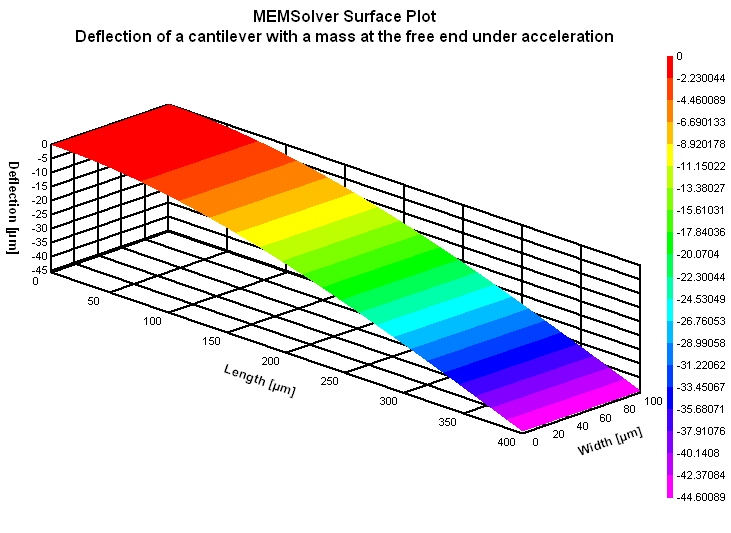MACLCANT,len,wid,thc,mas,acl,ymod,sel
Bending of a cantilever beam with a mass attached to the free end under acceleration load

len         length of the cantilever in µm
wid        width of the cantilever in µm
thc         thickness of the cantilever in µm
mas       mass at the free end in kg
acl         acceleration as multiples of g
an input value of 10 means 98.5m/s2
ymod     Young's modulus of the material of the beam in GPa
sel         number denoting the selected result.
Use 1 for maximum stress and 2 for maximum strain and 3 for maximum deflection of the beamNotes

Use this form to estimate the bending of a cantilever beam with an end mass due to an acceleration load. The acceleration is applied perpendicular to the plane of the beam. This is similar to an accelerometer. An acceleration load of 1g is equivalent to the weight of the mass acting on the beam. The maximum stress is at the top surface near the clamped edge and the maximum deflection is at the free end.

The X-Y plot shows the variation of maximum stress with change in the acceleration load. Using the crosshair, the maximum stress for any acceleration load from 0.1g to 100g can be calculated. The 2D and 3D surface plots show the deflection of the beam due to the load.Assumptions

-The default material is Silicon with a Young's modulus of 180GPa.
-The beam has uniform cross section.
-The bending of the mass is negligible.
-The deflection of the beam due to the weight of the mass is not considered.
-No other load acts on the structure other than the acceleration load perpendicular to the plane of the beam.
-Damping is not considered.